# Excel Tip: Do Not Disturb My Borders

How to copy a formula in Excel without changing borders.

A reader posed the following question: “I have built a report in Excel and used numerous borders to outline the data. After entering a formula to calculate profit in E3, I want to copy the formula down to E4 through E7 [Figure 1, below].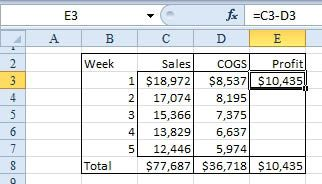However, because cell E3 has a top border, copying the formula causes all the cells in E4 through E7 to also have a top border, ruining the effect of my borders. [Figure 2, below].”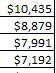Solution: You can select Home, Paste dropdown, Paste Special, All Except Borders to copy the formula and the numeric formatting but not disturb the borders (Figure 3).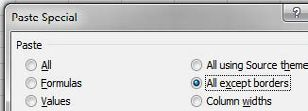The formula is successfully copied, but the borders remain as they were (Figure 4).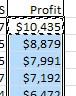Alternate Solution: In the data set described here, it appears that you decided to show the currency symbol on only the first row and the total row. In this case, it might have been more appropriate to use Paste Special, Formulas just to copy the formula. In Excel 2010, the Paste dropdown offers two icons in the first row that will help in this situation. The fx icon will copy formulas. The %fx icon will copy the formulas as well as number formatting (Figure 5).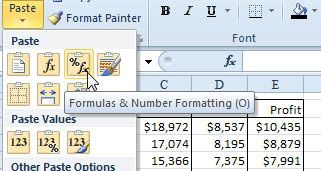CFO contributor Bill Jelen is an Excel MVP and the author of 35 books about Microsoft Excel. He is also editor-at-large of the CFO Excel Pro newsletter, where this article first appeared.